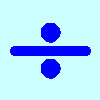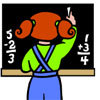# Resources tagged with: Divisibility

Filter by: Content type:
Age range:
Challenge level:

There are 41 NRICH Mathematical resources connected to Divisibility, you may find related items under Properties of Numbers.

Broad Topics > Properties of Numbers > Divisibility### Division Rules

##### Age 7 to 11Challenge Level

This challenge encourages you to explore dividing a three-digit number by a single-digit number.### Curious Number

##### Age 7 to 11Challenge Level

Can you order the digits from 1-3 to make a number which is divisible by 3 so when the last digit is removed it becomes a 2-figure number divisible by 2, and so on?### Going Round in Circles

##### Age 11 to 14Challenge Level

Mathematicians are always looking for efficient methods for solving problems. How efficient can you be?### The Remainders Game

##### Age 7 to 14Challenge Level

Play this game and see if you can figure out the computer's chosen number.##### Age 11 to 14Challenge Level

Powers of numbers behave in surprising ways. Take a look at some of these and try to explain why they are true.### Elevenses

##### Age 11 to 14Challenge Level

How many pairs of numbers can you find that add up to a multiple of 11? Do you notice anything interesting about your results?### Ben's Game

##### Age 11 to 16Challenge Level

Ben, Jack and Emma passed counters to each other and ended with the same number of counters. How many did they start with?### Peaches Today, Peaches Tomorrow...

##### Age 11 to 14Challenge Level

A monkey with peaches, keeps a fraction of them each day, gives the rest away, and then eats one. How long can his peaches last?### What an Odd Fact(or)

##### Age 11 to 14Challenge Level

Can you show that 1^99 + 2^99 + 3^99 + 4^99 + 5^99 is divisible by 5?### Take Three from Five

##### Age 11 to 16Challenge Level

Caroline and James pick sets of five numbers. Charlie chooses three of them that add together to make a multiple of three. Can they stop him?### Remainders

##### Age 7 to 14Challenge Level

I'm thinking of a number. My number is both a multiple of 5 and a multiple of 6. What could my number be?### Divisibility Tests

##### Age 11 to 16

This article explains various divisibility rules and why they work. An article to read with pencil and paper handy.### American Billions

##### Age 11 to 14Challenge Level

Play the divisibility game to create numbers in which the first two digits make a number divisible by 2, the first three digits make a number divisible by 3...### Neighbours

##### Age 7 to 11Challenge Level

In a square in which the houses are evenly spaced, numbers 3 and 10 are opposite each other. What is the smallest and what is the largest possible number of houses in the square?### Differences

##### Age 11 to 14Challenge Level

Can you guarantee that, for any three numbers you choose, the product of their differences will always be an even number?### Legs Eleven

##### Age 11 to 14Challenge Level

Take any four digit number. Move the first digit to the end and move the rest along. Now add your two numbers. Did you get a multiple of 11?### Dozens

##### Age 7 to 14Challenge Level

Can you select the missing digit(s) to find the largest multiple?### Big Powers

##### Age 11 to 16Challenge Level

Three people chose this as a favourite problem. It is the sort of problem that needs thinking time - but once the connection is made it gives access to many similar ideas.### Counting Factors

##### Age 11 to 14Challenge Level

Is there an efficient way to work out how many factors a large number has?### Multiple Surprises

##### Age 11 to 16Challenge Level

Sequences of multiples keep cropping up...### What Numbers Can We Make Now?

##### Age 11 to 14Challenge Level

Imagine we have four bags containing numbers from a sequence. What numbers can we make now?### What Numbers Can We Make?

##### Age 11 to 14Challenge Level

Imagine we have four bags containing a large number of 1s, 4s, 7s and 10s. What numbers can we make?### Gran, How Old Are You?

##### Age 7 to 11Challenge Level

When Charlie asked his grandmother how old she is, he didn't get a straightforward reply! Can you work out how old she is?### Flow Chart

##### Age 11 to 14Challenge Level

The flow chart requires two numbers, M and N. Select several values for M and try to establish what the flow chart does.### Digital Roots

##### Age 7 to 14

In this article for teachers, Bernard Bagnall describes how to find digital roots and suggests that they can be worth exploring when confronted by a sequence of numbers.### Three Times Seven

##### Age 11 to 14Challenge Level

A three digit number abc is always divisible by 7 when 2a+3b+c is divisible by 7. Why?### Just Repeat

##### Age 11 to 14Challenge Level

Think of any three-digit number. Repeat the digits. The 6-digit number that you end up with is divisible by 91. Is this a coincidence?### Remainder

##### Age 11 to 14Challenge Level

What is the remainder when 2^2002 is divided by 7? What happens with different powers of 2?### Divisively So

##### Age 11 to 14Challenge Level

How many numbers less than 1000 are NOT divisible by either: a) 2 or 5; or b) 2, 5 or 7?### Powerful Factorial

##### Age 11 to 14Challenge Level

6! = 6 x 5 x 4 x 3 x 2 x 1. The highest power of 2 that divides exactly into 6! is 4 since (6!) / (2^4 ) = 45. What is the highest power of two that divides exactly into 100!?### Eminit

##### Age 11 to 14Challenge Level

The number 8888...88M9999...99 is divisible by 7 and it starts with the digit 8 repeated 50 times and ends with the digit 9 repeated 50 times. What is the value of the digit M?##### Age 11 to 14Challenge Level

List any 3 numbers. It is always possible to find a subset of adjacent numbers that add up to a multiple of 3. Can you explain why and prove it?### Factoring Factorials

##### Age 11 to 14Challenge Level

Find the highest power of 11 that will divide into 1000! exactly.### Book Codes

##### Age 7 to 11Challenge Level

Look on the back of any modern book and you will find an ISBN code. Take this code and calculate this sum in the way shown. Can you see what the answers always have in common?### Gaxinta

##### Age 11 to 14Challenge Level

A number N is divisible by 10, 90, 98 and 882 but it is NOT divisible by 50 or 270 or 686 or 1764. It is also known that N is a factor of 9261000. What is N?### Digat

##### Age 11 to 14Challenge Level

What is the value of the digit A in the sum below: [3(230 + A)]^2 = 49280A### AB Search

##### Age 11 to 14 ShortChallenge Level

The five digit number A679B, in base ten, is divisible by 72. What are the values of A and B?### Square Routes

##### Age 11 to 14Challenge Level

How many four digit square numbers are composed of even numerals? What four digit square numbers can be reversed and become the square of another number?### Oh! Hidden Inside?

##### Age 11 to 14Challenge Level

Find the number which has 8 divisors, such that the product of the divisors is 331776.### Repeaters

##### Age 11 to 14Challenge Level

Choose any 3 digits and make a 6 digit number by repeating the 3 digits in the same order (e.g. 594594). Explain why whatever digits you choose the number will always be divisible by 7, 11 and 13.